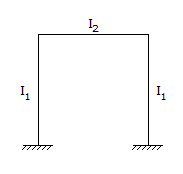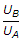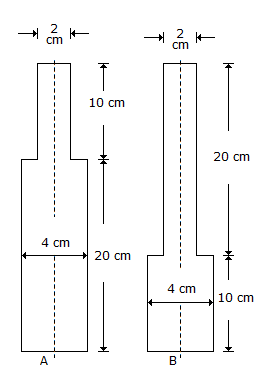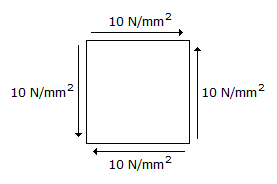# Civil Engineering - UPSC Civil Service Exam Questions

46.
The rigid portal frame shown in the given figure will not have any sidesway if
I1 = the moment of inertia of the column cross-section
I2 = the moment of inertia of the beam cross-sectionI2 = 2I1
Explanation:
No answer description is available. Let's discuss.

47.
Two similar round bars A and B are each 30 cm long as shown in the given figure. The ratio of the energies stored by the bars A and B, is3/2
1.0
5/8
2/3
Explanation:
No answer description is available. Let's discuss.

48.
Consider the following statements :
The effect of air entrainment in concrete is to
1. increase resistance to freezing and thawing.
2. improve workability.
3. decrease strength.
Which of these statements are correct ?
2 and 3
1 and 3
1 alone
1, 2 and 3
Explanation:
No answer description is available. Let's discuss.

49.
The state of stress at a point in a stressed element is shown in the given figure. The maximum tensile stress in the element will be20 N/mm2
lO2 N/mm2
10 N/mm2
zero